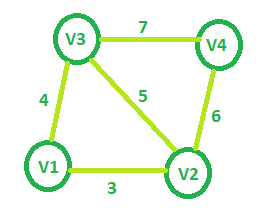# Minimum spanning tree cost of given Graphs

• Difficulty Level : Expert
• Last Updated : 16 Apr, 2021

Given an undirected graph of V nodes (V > 2) named V1, V2, V3, …, Vn. Two nodes Vi and Vj are connected to each other if and only if 0 < | i – j | ≤ 2. Each edge between any vertex pair (Vi, Vj) is assigned a weight i + j. The task is to find the cost of the minimum spanning tree of such graph with V nodes.
Examples:

Input: V = 4Output: 13
Input: V = 5
Output: 21

Approach: Starting with a graph with minimum nodes (i.e. 3 nodes), the cost of the minimum spanning tree will be 7. Now for every node i starting from the fourth node which can be added to this graph, ith node can only be connected to (i – 1)th and (i – 2)th node and the minimum spanning tree will only include the node with the minimum weight so the newly added edge will have the weight i + (i – 2)

So addition of fourth node will increase the overall weight as 7 + (4 + 2) = 13
Similarly adding fifth node, weight = 13 + (5 + 3) = 21
…
For nth node, weight = weight + (n + (n – 2))

This can be generalized as weight = V2 – V + 1 where V is the total nodes in the graph.
Below is the implementation of the above approach:

## C++

 `// C++ implementation of the approach` `#include ` `using` `namespace` `std;`   `// Function that returns the minimum cost` `// of the spanning tree for the required graph` `int` `getMinCost(``int` `Vertices)` `{` `    ``int` `cost = 0;`   `    ``// Calculating cost of MST` `    ``cost = (Vertices * Vertices) - Vertices + 1;`   `    ``return` `cost;` `}`   `// Driver code` `int` `main()` `{` `    ``int` `V = 5;` `    ``cout << getMinCost(V);`   `    ``return` `0;` `}`

## Java

 `// Java implementation of the approach ` `class` `GfG ` `{ `   `// Function that returns the minimum cost ` `// of the spanning tree for the required graph ` `static` `int` `getMinCost(``int` `Vertices) ` `{ ` `    ``int` `cost = ``0``; `   `    ``// Calculating cost of MST ` `    ``cost = (Vertices * Vertices) - Vertices + ``1``; `   `    ``return` `cost; ` `} `   `// Driver code ` `public` `static` `void` `main(String[] args) ` `{ ` `    ``int` `V = ``5``; ` `    ``System.out.println(getMinCost(V)); ` `}` `} `   `// This code is contributed by ` `// Prerna Saini.`

## C#

 `// C# implementation of the above approach ` `using` `System;`   `class` `GfG ` `{ `   `    ``// Function that returns the minimum cost ` `    ``// of the spanning tree for the required graph ` `    ``static` `int` `getMinCost(``int` `Vertices) ` `    ``{ ` `        ``int` `cost = 0; ` `    `  `        ``// Calculating cost of MST ` `        ``cost = (Vertices * Vertices) - Vertices + 1; ` `    `  `        ``return` `cost; ` `    ``} ` `    `  `    ``// Driver code ` `    ``public` `static` `void` `Main() ` `    ``{ ` `        ``int` `V = 5; ` `        ``Console.WriteLine(getMinCost(V)); ` `    ``}` `} `   `// This code is contributed by Ryuga`

## Python3

 `# python3 implementation of the approach` ` `  `# Function that returns the minimum cost` `# of the spanning tree for the required graph` `def` `getMinCost( Vertices):` `    ``cost ``=` `0` ` `  `    ``# Calculating cost of MST` `    ``cost ``=` `(Vertices ``*` `Vertices) ``-` `Vertices ``+` `1` ` `  `    ``return` `cost` ` `  `# Driver code` `if` `__name__ ``=``=` `"__main__"``:`   `    ``V ``=` `5` `    ``print` `(getMinCost(V))`

## PHP

 ``

## Javascript

 ``

Output:

`21`

My Personal Notes arrow_drop_up
Recommended Articles
Page :